# Samacheer Kalvi Books: Tamilnadu State Board Text Books Solutions

## Samacheer Kalvi 11th Computer Science Chapter 2 Number Systems Notes PDF Download: Tamil Nadu STD 11th Computer Science Chapter 2 Number Systems NotesSamacheer Kalvi 11th Computer Science Chapter 2 Number Systems Notes PDF Download: Tamil Nadu STD 11th Computer Science Chapter 2 Number Systems Notes

## Samacheer Kalvi 11th Computer Science Chapter 2 Number Systems Notes PDF Download

We bring to you specially curated Samacheer Kalvi 11th Computer Science Chapter 2 Number Systems Notes PDF which have been prepared by our subject experts after carefully following the trend of the exam in the last few years. The notes will not only serve for revision purposes, but also will have several cuts and easy methods to go about a difficult problem.

 Board Tamilnadu Board Study Material Notes Class Samacheer Kalvi 11th Computer Science Subject 11th Computer Science Chapter Chapter 2 Number Systems Format PDF Provider Samacheer Kalvi Books

## How to Download Samacheer Kalvi 11th Computer Science Chapter 2 Number Systems Notes PDFs?

2. Click on the Samacheer Kalvi 11th Computer Science Notes PDF.
3. Look for your preferred subject.
4. Now download the Samacheer Kalvi 11th Computer Science Chapter 2 Number Systems notes PDF.

## Download Samacheer Kalvi 11th Computer Science Chapter 2 Number Systems Chapterwise Notes PDF

PART – 1

11th Computer Science Chapter 2 Book Back Answers Question 1.
Which refers to the number of bits processed by a computer’s CPU?
(a) Byte
(b) Nibble
(c) Word length
(d) Bit
(c) Word length

11th Computer Science Chapter 2 Question 2.
How many bytes does 1 KiloByte contain?
(a) 1000
(b) 8
(c) 4
(d) 1024
(d) 1024

Class 11 Computer Science Chapter 2 Question Answer Question 3.
Expansion for ASCII ………………..
(а) American School Code for Information Interchange
(b) American Standard Code for Information Interchange
(c) All Standard Code for Information Interchange
(d) American Society Code for Information Interchange
(b) American Standard Code for Information Interchange

11th Computer Science 2nd Lesson Book Back Answers Question 4.
2^50 is referred as
(a) Kilo
(b) Tera
(c) Peta
(d) Zetta
(c) Peta

Samacheer Kalvi Guru 11th Computer Science Question 5.
How many characters can be handled in Binary Coded Decimal System?
(a) 64
(b) 255
(c) 256
(d) 128
(a) 64

11th Computer Science 2nd Lesson Question 6.
For 11012 what is the Hexadecimal equivalent?
(a) F
(b) E
(c) D
(d) B
(c) D

Questions On Number System Class 11 Computer Science Question 7.
What is the 1’s complement of 00100110?
(a) 00100110
(b) 11011001
(c) 11010001
(d) 00101001
(b) 11011001

Class 11 Computer Science Chapter 2 Pdf Question 8.
Which amongst this is not an Octal number?
(a) 645
(b) 234
(c) 876
(d) 123
(c) 876

Class 11 Computer Science Chapter 2 Solutions Question 1.
What is data?
The data is a fact about people, places, or some object. In a program, a value assigned to a variable is called data.

Samacheer Kalvi Guru 11 Computer Science Question 2.
Write the 1’s complement procedure.
Step 1: convert given decimal number into Binary
Step 2: if the binary bit contains 8 bits if less add 0 at the left most bit, to make it 8 bits.
Step 3: Invert all the bits, (i.e.) change 1 as 0 and 0 as 1.

Samacheer Kalvi.Guru 11th Computer Science Question 3.
Convert (46)10 into a Binary number
(46)10 into binary = 1011102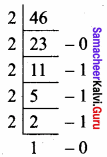Computer Science Chapter 2 Question 4.
We cannot find 1’s complement for (28)10. State reason.
Complement is only for negative numbers. (28)10 is a positive number. So we can’t find 1’s complement.

Class 11 Computer Science Chapter 2 Notes Question 5.
List the encoding systems for characters in memory.

1. BCD – Binary Coded Decimal
2. EBCDIC – Extended Binary Coded Decimal Interchange Code
3. ASCII – American Standard Code for Information Interchange
4. Unicode
5. ISCII – Indian standard code for information interchange

III. Explain in Brief

Question 1.
What is the radix of a number system? Give example.
The radix refers to the base of a number system: the total number of possible digits. The decimal number system that we all use is base ten, as it has ten distinct digits (0, 1, 2, 3, 4, 5, 6, 7, 8, 9).
Example: Binary system – Radix 2

Question 2.
Write a note on the binary number system.
In Binary Number System, there are only two digits namely 0 and 1. The numbers in the binary system are represented to the base 2. In the Binary Number, the leftmost bit is the Most Significant Bit (MSB) and the rightmost bit is the Least Significant Bit (LSB). MSB has largest positional weight and LSB has the smallest positional weight.
Example: 1100102

Question 3.
Convert (150)10 into Binary, then convert that Binary number to Octal.
Step 1:
Change it to Binary.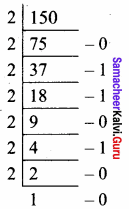(150)10 = 10010110

Step – 2:
Change it to Octal.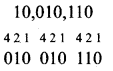Binary 100101102
Octal 226g

Question 4.
Write a short note on ISCII.
Indian Standard Code for Information Interchange (ISCII).
ISCII is the system of handling the character of Indian local languages. This is an 8-bit coding system. Therefore it can handle 256 (28) characters.

The supported scripts are:
Assamese, Bengali (Bangla), Devanagari, Gujarati, Gurmukhi, Kannada, Malayalam, Oriya, Tamil, and Telugu. ISCII does not encode the writing systems of India based on Arabic, but its writing system switching codes none the less provide for Kashmiri, Sindhi, Urdu, Persian, Pashto and Arabic. The Arabic – based writing systems were subsequently encoded in the PASCII encoding.

ISCII is an 8 – bit encoding. The lower 128 code points are plain ASCII, the upper 128 code points are ISCII – specific. In addition to the code points representing characters, ISCII makes use of a code point with mnemonic ATR that indicates that the following byte contains one of two kinds of information. One set of values changes the writing system until the next writing system indicator or end – of – line.

Question 5.
Add (a) – 2210 + 1510
(b) 2010 + 2510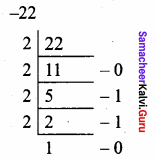(a) – 2210 = 101102
Binary Equivalent = 101102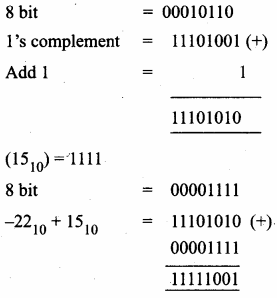111110012

(b) 2010 + 2510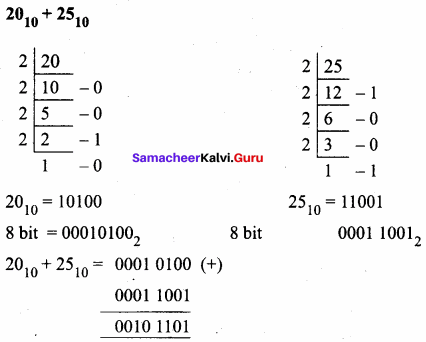001011012

Question 1.
(a) Write the procedure to convert fractional Decimal to Binary.
(b) Convert (98.46)10 to Binary
(а) Procedure to convert fractional Decimal to Binary.
Step 1: Multiply the decimal fraction by 2 and note the integer part. The integer part is either 0 or 1.
Step 2: Discard the integer part of the previous product. Multiply the fractional part of the previous product by 2. Repeat step 1 until the same fraction repeats or terminates (0).
Step 3: The resulting integer part forms a sequence of 0’s and 1 ’s that becomes the binary equivalent of a decimal fraction.
Step 4: The final answer is to be written from the first integer part obtained till the last integer part obtained.

(b) Convert (98.46)10 to Binary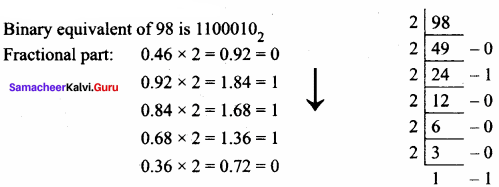1100010.01110 … 2

Question 2.
Find 1’s Complement and 2’s Complement for the following Decimal number
(a) -98
(b) -135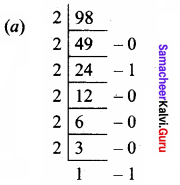9810 = 01100010
8 bit format = 01100010
1’s complement = 10011101
Add 1 bit = +1 = 10011110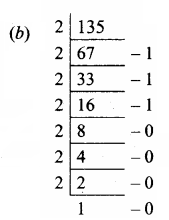13510 = 10000111
1’st complement = 01111000
Add 1 bit = + 1
= 01111001

Question 3.
(b) Subtract 11010112 – 1110102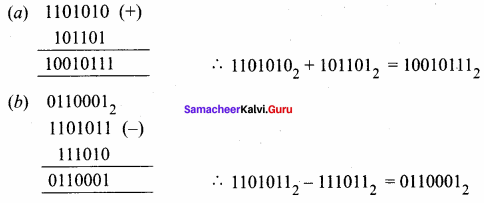PART – 1

Question 1.
Which is a basic electronic circuit which operates on one or more signals?
(a) Boolean algebra
(b) Gate
(c) Fundamental gates
(d) Derived gates
(b) Gate

Question 2.
Which gate is called the logical inverter?
(a) AND
(b) OR
(c) NOT
(d) XNOR
(c) NOT

Question 3.
A + A = ?
(a) A
(b) O
(c) 1
(d) A
(a) A

Question 4.
NOR is a combination of?
(a) NOT(OR)
(b) NOT(AND)
(c) NOT(NOT)
(d) NOT(NOR)
(a) NOT(OR)

Question 5.
NAND is called as ……………… Gate.
(a) Fundamental Gate
(b) Derived Gate
(c) Logical Gate
(d) Electronic gate
(b) Derived Gate

PART – 2

Question 1.
What is Boolean Algebra?
Boolean algebra is a mathematical discipline that is used for designing digital circuits in a digital computer. It describes the relationship between inputs and outputs of a digital circuit.

Question 2.
Write a short note on NAND Gate.
The NAND gate is the combination of NOT and AND gates. The NAND gate is generated by inverting the output of a AND gate. The algebraic expression of the NAND is Y = A.B¯¯¯¯¯¯¯¯¯

Question 3.
Draw the truth table for the XOR gate.
The truth table for XOR gate is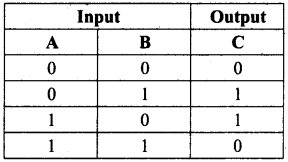Question 4.
Write the associative laws?
Associative Laws:
i) A + (B + C) = (A + B) + C
ii) A . (B . C) = (A . B) . C

Question 5.
What are derived gates?
Derived Gates are the gates that are derived from the fundamental gates.
Example: NAND, NOR, XOR, XNOR

PART – 3
III. Explain in Brief

Question 1.
Write the truth table of fundamental gates.
(a) AND gate – Truth Table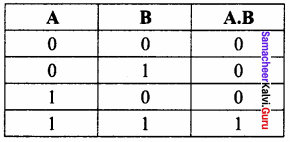(b) OR gate – Truth Table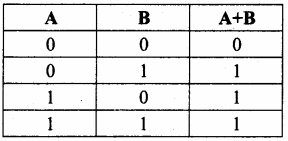(c) NOT Gate – Truth Table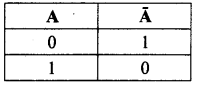Question 2.
Write a short note on XNOR gate.
The XNOR (exclusive – NOR) gate is a combination of XOR gate followed by an inverter. Its output is “true” if the inputs are same and false if the inputs are different.
The output of XNOR is C = AB + A¯¯¯¯ B¯¯¯¯
= A B
Where indicates included dot.
Truth Table: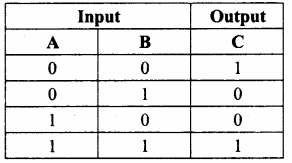Logic symbol: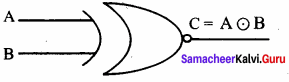Logic circuit: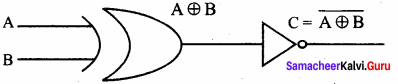Question 3.
Reason out why the NAND and NOR are called universal gates?
NAND and NOR gates are called Universal gates because the fundamental logic gates can be realized through them.

Question 4.
Give the truth table of XOR gate.
XOR – Truth Table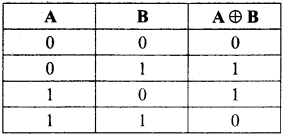Question 5.
Write the De Morgan’s law.
De Morgan’s laws
i)A+B¯¯¯¯¯¯¯¯¯¯¯¯¯=A¯¯¯¯B¯¯¯¯ii)(A¯B)=A¯+B¯

PART – 4
IV. Explain in Detail

Question 1.
Explain the fundamental gates with an expression and truth table.
the gate is a basic electronic circuit that operates on one or more signals to produce an output signal.
The three fundamental gates are AND, OR, and NOT gates.

AND Gate:
The AND gate can have two or more input signals and produce an output signal. The output is “true” only when both inputs are “true”, otherwise, the output is “false”. In other words, the output will be 1 if and only if both inputs are 1; otherwise, the output is 0. The output of the AND gate is represented by a variable say C, where A and B are two and if input boolean variables. In boolean algebra, a variable can take either of the values ‘0’ or ‘1’. The logical symbol of the AND gate is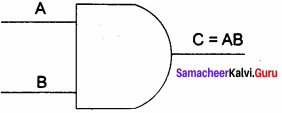The truth table for AND gate is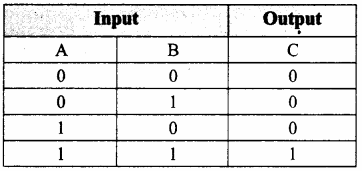OR Gate:
The OR gate gets its name from its behaviour like the logical inclusive “OR”. The output is “true” if either or both of the inputs are “true”. If both inputs are “false” then the output is “false”. In other words the output will be 1 if and only if one or both inputs are 1; otherwise, the output is 0. The logical symbol of the OR gate is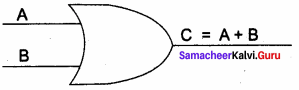The truth table for OR gate is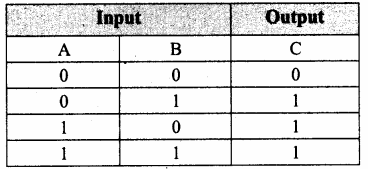NOT Gate:
The NOT gate, called a logical inverter, has only one input. It reverses the logical state. In other words, the output C is always the complement of the input. The logical symbol of the NOT gate is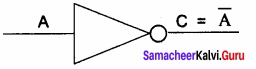The truth table for NOT gate is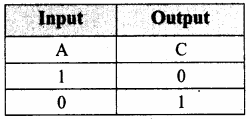Question 2.
How AND and OR can be realized using NAND and NOR gate.
Bubbled AND Gate
(i) Realized of and using only AND gate:
The Boolean function for AND is C = AB. The same can be realized using only NAND gates.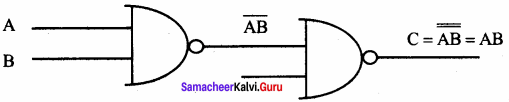(ii) Realization of or using only NAND’s:
The Boolean function of OR is C = A + B. The same can be realized using only NAND gates.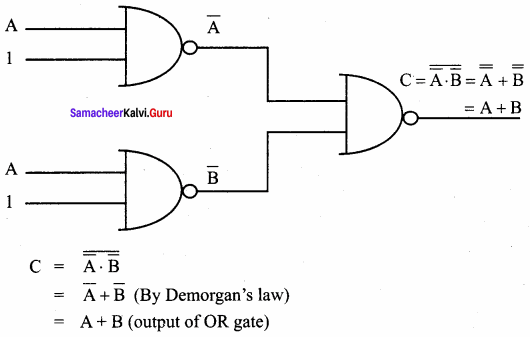(iii) Realization of AND using NOR:
By using only the NOR gates, we can get the output equivalent to the output of AND gate.
C = A.B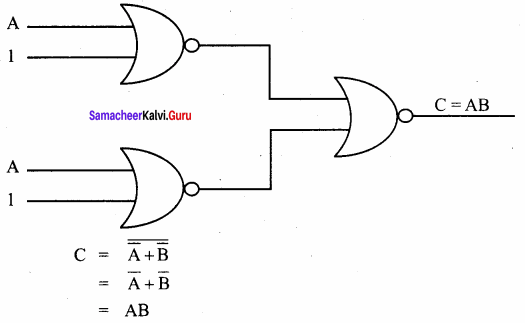(iv) Realization of OR using NOR’s:
By using only NOR gates we are getting the output equivalent to OR gate.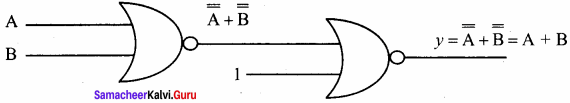Question 3.
Explain the Derived gates with expression and truth table.
The logic gates like NAND, NOR, XOR, and XNOR are derived gates which are derived from the fundamental gates AND, OR NOT.

(i) NAND gate:
The NAND is the combination of NOT and AND. The NAND is generated by inverting the output of an AND operation.
Output: y = AB¯¯¯¯¯¯¯
Logic circuit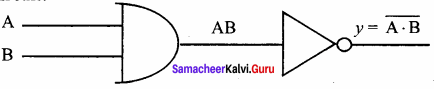Logic symbol: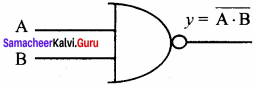Truth table: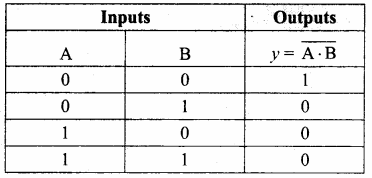Inputs Outputs
(ii) NOR gate:
The NOR is the combination of NOT and OR. The NOR is generated by inverting the output of an OR operation.
Logic function: y = AB¯¯¯¯¯¯¯
Logic circuit: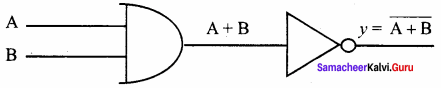logic symbol: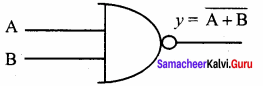Truth table: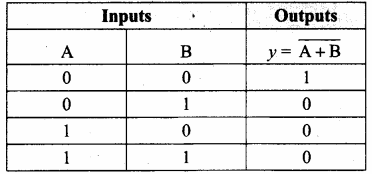(iii) XOR gate:
The XOR (exclusive – OR) gate acts in the same way as the logical either /or.
Logic symbol: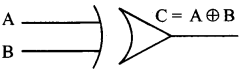Logic circuit: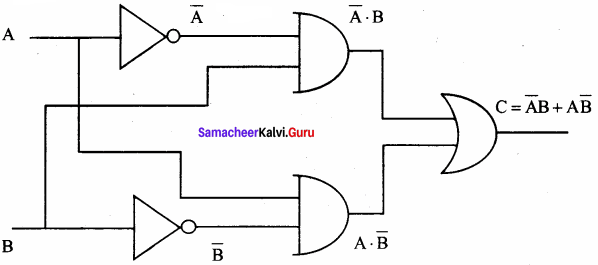Truth table: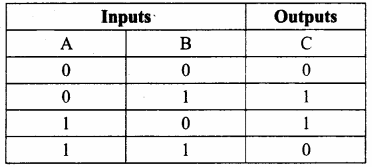(iv) XNOR gate:
XNOR gate (exclusive – NOR) gate is a combination of XOR gate followed by an inverter.
Logic function: y = AB¯¯¯¯¯¯¯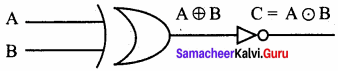logic symbol: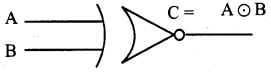Truth table: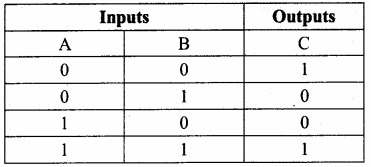Boolean function: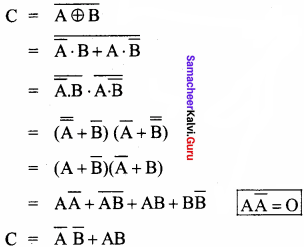### Samacheer Kalvi 11th Computer Science Number Systems Additional Questions and Answers

PART – 1

Question 1.
Computer handles data in the form of ________________
a) 1
b) 0
c) alphabets
d) both A and B
d) both A and B

Question 2.
The term data comes from the word ………………..?
(a) number
(b) datum
(c) nibble
(d) bit
(b) datum

Question 3.
0 and 1 are called________________
a) BIT
b) BYTE
c) NIBBLE
d) WORD
a) BIT

Question 4.
……………….. scheme is denoted by hexadecimal numbers.
(a) binary
(b) Unicode
(c) word length
(d) data
(b) Unicode

Question 5.
A_________ is the short form of Binary digit.
a) BIT
b) BYTE
c) NIBBLE
d) WORD
a) BIT

Question 6.
A ……………….. number is represented using base 16.
(b) octal
(c) binary
(d) decimal

Question 7.
A________ is a collection of 4 bits.
a) BIT
b) BYTE
c) NIBBLE
d) WORD
c) NIBBLE

Question 8.
Octal number system uses digits ………………..
(a) 7
(b) 5
(c) 8
(d) 10
(c) 8

Question 9.
The memory size of the computer is measured in the unit called __________
a) BIT
b) BYTE
c) NIBBLE
d) WORD
b) BYTE

Question 10.
The NAND gate operates an AND gate followed by a ……………….. gate.
(a) AND
(b) OR
(c) NOT
(d) XOR
(c) NOT

Question 11.
The word length of the present day computer is __________ bits.
a) 32
b) 64
c) 8
d) 32 or 64
d) 32 or 64

Question 12.
Expand BIT.
(a) Basic Input Term
(b) Binary Input Term
(c) Binary Digit
(d) Binary Inverse Digit
(c) Binary Digit

Question 13.
_________is not a valid word length of a computer.
a) 8
b) 12
c) 4
d) both B and C
d) both B and C

Question 14.
Identify the wrong pair.
1. 1 YB = 2^80
2. 1 BM = 2^70
3. 1 MB = 2^20
4. 1 TM = 2^40
(a) 2
(b) 3
(c) 4
(d) 1
(a) 2

Question 15.
1 MegaByte equals t______ KiloBytes.
a) 1024
b) 256
c) 1000
d) 128
a) 1024

Question 16.
The collection of 4 bits is ………………..
(a) bit
(b) byte
(c) nibble
(d) KB
(c) nibble

Question 17.
Match the following.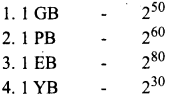(a) 4 12 3
(b) 12 3 4
(c) 4 3 2 1
(d) 4 2 1 3
(a) 4 12 3

Question 17.
1024 GigaByte equals to
a) 1 GigaByte
b) 1 TeraByte
c) 1 YottaByte
d) None of these
b) 1 TeraByte

Question 19.
1 MB equals to ________ bytes.
a) 210
b) 220
c) 230
d) 240
b) 220

Question 20.
What is the decimal value of 11112?
(a) 10
(b) 11
(c) 14
(d) 15
(d) 15

Question 21.
1 TB equals to_______ bytes
a) 210
b) 220
c) 230
d) 240
d) 240

Question 22.
The decimal value of Binary number 10 is ………………..
(a) 101010
(b) 2
(c) 100
(d) A
(b) 2

Question 23.
1 ExaByte (1EB) equals to _________ bytes
a) 250
b) 260
c) 270
d) 280
b) 260

Question 24.
Which of the following are data?
(a) Alphabet
(b) Special character
(c) Number
(d) All of these
(d) All of these

Question 25.
1 YottaByte equals to_________ bytes.
a) 250
b) 260
c) 270
d) 280
d) 280

Question 26.
Pick the odd one.
(a) BCD
(b) ENIAC
(c) ASCII
(d) EBCDIC
(b) ENIAC

Question 27.
________are used to represent characters in a text.
a) Bytes
b) Bits
c) Nibbles
d) None of these
a) Bytes

Question 28.
Unicode can handles how many characters?
(a) 64
(b) 128
(c) 256
(d) 65536
(d) 65536

Question 29.
ASCII is a _________ bit code.
a) 8
b) 7
c) 16
d) 32
b) 7

Question 30.
Which one is the rightmost bit?
(a) MSB
(b) LSB
(c) USB
(d) USRB
(b) LSB

Question 31.
The ASCII value for Numeric 0(zero) is_______
a) 48
b) 38
c) 128
d) 32
a) 48

Question 32.
The leftmost bit of a positive binary number in signed notation is ………………..
(a) 0
(b) 1
(c) 2
(d) A
(a) 0

Question 33.
Each number system is uniquely identified by its _________
a) base value
d) symbols

Question 34.
The radix for the octal number system is ………………..
(a) 2
(b) 8
(c) 1
(d) 16
(b) 8

Question 35.
_________is the general idea behind positional numbering system.
a) base value
d) symbols

Question 36.
Which one of the following companies have formulated EBCDIC?
(a) Microsoft
(b) 1 BM
(c) Sun
(d) Apple
(b) 1 BM

Question 37.
The leftmost bit in the binary number is called the _________
a) MSB
b) LSB
c) FSB
d) None of these
a) MSB

Question 38.
The base value of a hexadecimal number is ………………..
(a) 2
(b) 8
(c) 16
(d) 18
(c) 16

Question 39.
Each octal digit has its own positional value or weight as a power of _________
a) 8
b) 16
c) 4
d) 10
a) 8

Question 40.
How many truth values are there?
(a) 2
(b) 3
(c) 4
(d) 5
(a) 2

Question 41.
_______system is used to represent data in a more compact manner.
b) Octal
c) Decimal
d) None of these

Question 42.
The variables which can store the truth values are called………………..
(a) logical variable
(b) binary-valued variable
(c) boolean variables
(d) all of these
(d) all of these

Question 43.
How many methods are there to convert decimal numbers to binary numbers?
a) only one
b) 2
c) 3
d) many
b) 2

Question 44.
Which is not a logical operator?
(a) dot
(b) plus
(c) over bar
(d) command
(d) command

Question 45.
Computer can handle _______numbers.
a) positive
b) negative
c) positive and negative
d) None of these
c) positive and negative

Question 46.
Which symbol is used in the OR operator?
(a) –
(b) •
(c) *
(d) +
(d) +

Question 47.
The simplest method to represent negative binary numbers is called_______method.
a) 1’s complement
b) 2’s complement
c) signed magnitude
d) All the above
c) signed magnitude

Question 48.
Which among the following can be replaced by a bubbled AND gate?
(a) AND
(b) NAND
(c) OR
(d) not
(b) NAND

Question 49.
How many ways a number can be represented in computers?
a) 3
b) 2
c) only one
d) None of these
a) 3

Question 50.
Find the universal gates from the following.
(a) XOR
(b) XNOR
(c) a and b
(d) NOR
(d) NOR

Question 51.
If the number has _______sign, it will be considered as positive in signed magnitude representation.
a) +
b) no
c) + or ++
d) A or B
d) A or B

Question 52.
Which symbol is used in the XOR gate?
(a)
(b)
(c)
(d) –
(c)

Question 53.
Included dot means ………………..
(a)
(b) [•]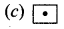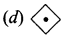(a)

Question 53.
1’s complement of (00011000)2 is _______
a) 11100111
b) 00011001
c) 00000011
d) None of these
a) 11100111

Question 55.
Identify the statement which is wrong.
(a) A . 1 = A
(b) A . A = A
(c) A + O = A
(d) A . 1 = 0
(b) A . A = A

Question 55.
The binary numbers permits _______ computation.
b) multiplication
c) division
d) all the above
d) all the above

Question 57.
Identify the statements which are true.
(i) A + 0 = A
(ii) A.A = A
(iii) A + A¯¯¯¯ = 1
(iv) A. O = O

(a) (iii) (iv) are true
(b) (i) (ii) are true
(c) (i) (ii) (iii) are true.
(d) all are true
(d) all are true

Question 58.
All the characters in the character set are denoted through_______only.
a) numbers
b) alphanumeric
c) alphabet
d) None of these
a) numbers

Question 59.
Match the following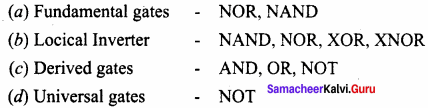(a) 3 4 2 1
(b) 1 2 3 4
(c) 4 3 2 1
(d) 3 4 1 2
(a) 3 4 2 1

Question 60.
BCD stands for _______
a) Binary Coded Decimal
b) Binary Character Decoding
c) Basic Coded Decimal
d) Bit Coded Decimal
a) Binary Coded Decimal

PART – 2

Question 1.
Define data.
Data is an unprocessed collection of facts given as input to the computer. In a program, the values assigned to the variables are called data.

Question 2.
Expand ASCII.
American Standard Code for Information Interchange

Question 3.
What do you mean by nibble?
A nibble is a collection of 4 bits binary digits.

Question 4.
What is MSB and LSB?
MSB means Most Significant Bit; LSB-Least Significant Bit

Question 5.
What do you mean by word length?
Word length refers to the number of bits processed by a Computer’s CPU. For example, a word length can have 8 bits, 16 bits, 32 bits, and 64 bits.

Question 6.
Define word length?
Word length refers to the number of bits processed by a computer’s CPU.

Question 7.
Write note on number system.
A numbering system is a way of representing numbers. The most commonly used numbering system in real life is the Decimal number system. Other number systems are Binary, Octal, and Hexadecimal Number System.

Question 8.
How will you convert a number from octal to Binary?
For each octal digit in the given number write its 3 digits binary equivalent using positional notation.

Question 9.
Why the computers are working at different speeds?
The speed of a computer depends on the number of bits it can process at once. For example, a 64- bit computer can process 64-bit numbers in one operation, while a 32-bit computer breaks 64- bit numbers down into smaller pieces, making it slower.

Question 10.
Write a short note on BCD.

1. BCD stands for Binary Coded Decimal system.
2. BCD is a 26-bit encoding system.
3. IT can handle 64 characters.

PART – 3
III. Explain in Brief

Question 1.
What is a binary number system?
There are only two digits in the Binary system, namely, 0 and 1. The numbers in the binary system are represented to the base 2 and the positional multipliers are the powers of 2. The leftmost bit in the binary number is called as the Most Significant Bit (MSB) and it has the largest positional weight. The rightmost bit is the Least Significant Bit (LSB) and has the smallest positional weight.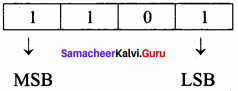Example:
The binary sequence (11101)2 has the decimal equivalent:
(1101)2 = 1 x 23 + 1 x 22 + 0 x 21 + 1 x 20
= 8 + 4 + 0 + 1
= (13)10

Question 2.
A hexadecimal number is represented using base 16. Hexadecimal or Hex numbers are used as a shorthand form of a binary sequence. This system is used to represent data in a more compact manner. Since 16 symbols are used, 0 to F, the notation is called hexadecimal.

The first 10 symbols are the same as in the decimal system, 0 to 9, and the remaining 6 symbols are taken from the first 6 letters of the alphabet sequence, A to F, where A represents 10, B is 11, C is 12, D is 13, E is 14 and F is 15.

Question 3.
What is a decimal number system?
It consists of 0, 1, 2, 3, 4, 5, 6, 7, 8, 9(10 digits). It is the oldest and most popular number system used in our day to day life. In the positional number system, each decimal digit is weighted relative to its position in the number. This means that each digit in the number is multiplied by 10 raised to a power corresponding to that digit’s position.
Example:
(123)10 = (1 x 102) + (2 x 101) + (3 x 100)
= 100 + 20 + 3
= (123)10

Question 4.
Write the distributive law.
A . (B + C) = A . B + A . C
A + (B . C) = (A + B) . (A + C)

Question 5.
What is the truth table?
A truth table represents all the possible values of logical variables or statements along with all the possible results of a given combination of truth values.

PART – 4
IV. Explain in Detail

Question 1.
Explain the different types of number systems?
Different Types of Number Systems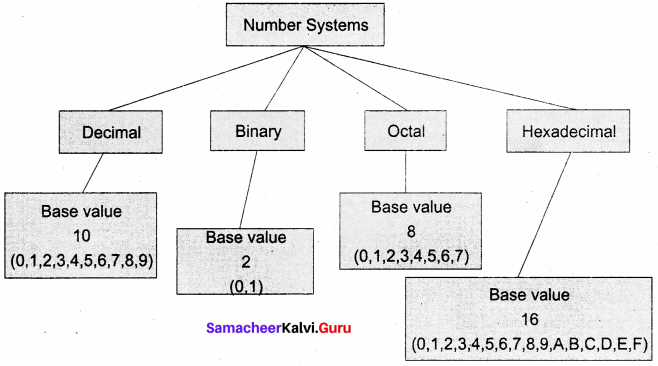A numbering system is a way of representing numbers. The most commonly used numbering system in real life is the Decimal number system. Other number systems are the Binary, Octal, Hexadecimal number systems. Each number system is uniquely identified by its base value or radix. Radix or base is the count of a number of digits in each number system. Radix or base is the general idea behind the positional numbering system.

Decimal Number System:
It consists of 0, 1, 2, 3, 4, 5, 6, 7, 8, 9(10 digits). It is the oldest and most popular number system used in our day-to-day life. In the positional number system, each decimal digit is weighted relative to its position in the number. This means that each digit in the number is multiplied by 10 raised to a power corresponding to that digit’s position.
Example:
(123)10 = 1 x 102 + 2 x 101 + 3 x 100
= 100 + 20 + 3
= (123)10

Binary Number System:
There are only two digits in the Binary system, namely, 0 and 1. The numbers in the binary system are represented to the base 2 and the positional multipliers are the powers of 2. The leftmost bit in the binary number is called the Most Significant Bit (MSB) and it has the largest positional weight. The rightmost bit is the Least Significant Bit (LSB) and has the smallest positional weight.Example:
The binary sequence (1101)2 has the decimal equivalent:
(1101)2 = 1 x 23 + 1 x 22 + 0 x 21 + 1 x 20
= 8 + 4 + 0 + 1
= (13)10

Octal Number System:
Octal number system uses digits 0,1,2,3,4,5,6 and 7 (8 digits). Each octal digit has its own positional value or weight as a power of 8.
Example:
The Octal sequence (547)8 has the decimal equivalent:
(547)8 = 5 x 82 + 4 x 82 + 7 x 80
= 5 x 64 + 4 x 8 + 7 x 1
= 320 + 32 + 7
= (359)10

A hexadecimal number is represented using base 16. Hexadecimal or Hex numbers are used as a shorthand form of a binary sequence. This system is used to represent data in a more compact manner. Since 16 symbols are used, 0 to F, the notation is called hexadecimal. The first 10 symbols are the same as in the decimal system, 0 to 9, and the remaining 6 symbols are taken from the first 6 letters of the alphabet sequence, A to F, where A represents 10, B is 11, C is 12, D is 13, E is 14 and F is 15.

Question 2.
Explain the octal to decimal conversion and hexadecimal to decimal conversion.
Octal to Decimal Conversion:
To convert Octal to Decimal, we can use the positional notation method.

1. Write down the Octal digits and list the powers of 8 from right to left(Positional Notation).
2. For each positional notation of the digit write the equivalent weight.
3. Multiply each digit with its corresponding weight.

Example:
Convert (1265)8 to an equivalent Decimal number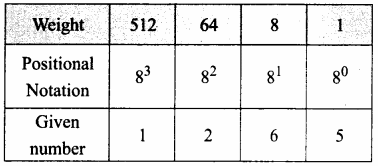(1265)8 = 512 x 1 + 64 x 2 + 8 x 6 + 1 x 5
= 512 + 128 + 48 + 5
(1265)8 = (693)10

To convert Hexadecimal to Decimal we can use the positional notation method.

1. Write down the Hexadecimal digits and list the powers of 16 from right to left (Positional Notation).
2. For each positional notation written for the digit, now write the equivalent weight.
3. Multiply each digit with its corresponding weight.
4. Add all the values to get one final value.

Example:
Convert (25F)16 into its equivalent Decimal number.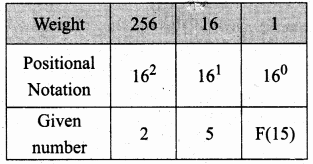Question 3.
Explain the binary addition and binary subtraction
The following table is useful when adding two binary numbers.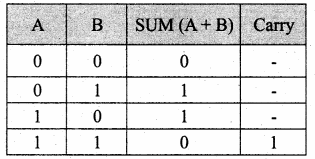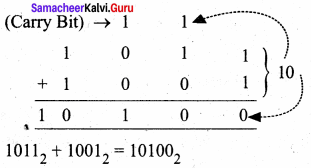Example: Perform Binary addition for the following:
2310 + 1210
Step 1: Convert 23 and 12 into binary form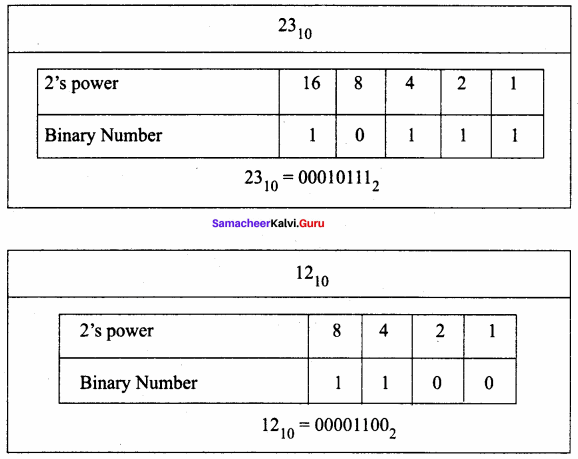Step 2: Binary addition of 23 and 12: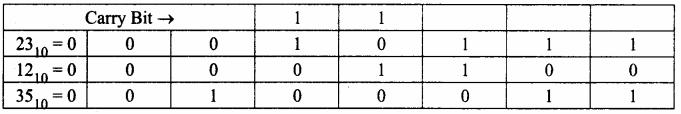Binary Subtraction:
The table for Binary Subtraction is as follows: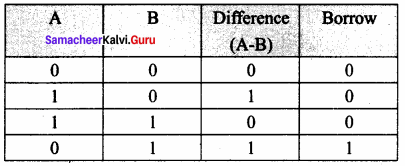When subtracting 1 from 0, borrow 1 from the next Most Significant Bit, when borrowing from the next Most Significant Bit, if it is 1, replace it with 0. If the next Most Significant Bit is 0, you must borrow from a more significant bit that contains 1 and replace it with 0, and 0s upto that point become 1’s.
Example: Subtract 10010102 – 101002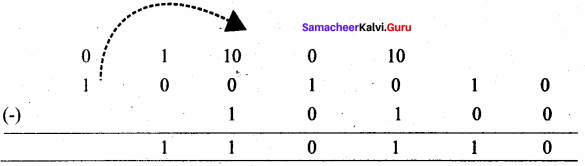Example Perform binary addition for the following: (-21)10 + (5)10
Step 1: Change – 21 and 5 into binary form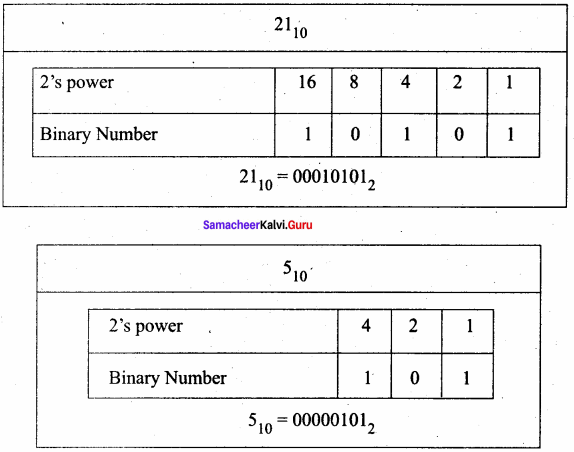Step 2: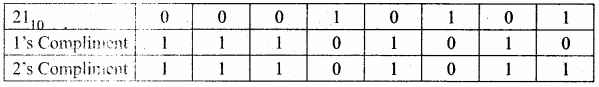Step 3:
Binary Addition of – 21 and 5: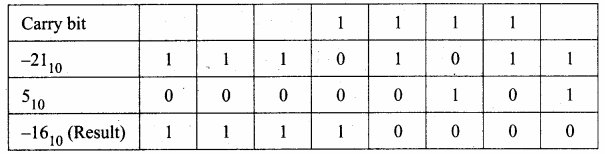Question 4.
Explain the theorems of boolean algebra.
Theorems of Boolean Algebra:
Identity:
A + 0 = A
A. 1 = A

Complement:
A + A¯¯¯¯ = 1
A. A¯¯¯¯ = 0

Commutative:
A + B = B + A
A. B = B .A

Associative:
A + (B + C) = (A + B) + C
A. (B . C) = (A. B). C

Distributive
A. (B + C) = A B + A. C
A + (B . C) = (A + B). (A + C)

Null Element:
A + 1 = 1
A. 0 = 0

Involution
(A=) = A

Indempotence:
A +A = A
A.A = A

Absorption:
A + (A . B) = A
A . (A + B) = A

3rd Distributive:
A + A¯¯¯¯. B = A + B

De Morgan’s:
A+B¯¯¯¯¯¯¯¯¯¯¯¯¯ = A¯¯¯¯.B¯¯¯¯
(A.B¯¯¯¯¯¯¯¯¯) = A¯¯¯¯ + B¯¯¯¯

## How to Prepare using Samacheer Kalvi 11th Computer Science Chapter 2 Number Systems Notes PDF?

Students must prepare for the upcoming exams from Samacheer Kalvi 11th Computer Science Chapter 2 Number Systems Notes PDF by following certain essential steps which are provided below.

• Use Samacheer Kalvi 11th Computer Science Chapter 2 Number Systems notes by paying attention to facts and ideas.
• Pay attention to the important topics
• Refer TN Board books as well as the books recommended.
• Correctly follow the notes to reduce the number of questions being answered in the exam incorrectly
• Highlight and explain the concepts in details.

## Frequently Asked Questions on Samacheer Kalvi 11th Computer Science Chapter 2 Number Systems Notes

#### How to use Samacheer Kalvi 11th Computer Science Chapter 2 Number Systems Notes for preparation??

Read TN Board thoroughly, make separate notes for points you forget, formulae, reactions, diagrams. Highlight important points in the book itself and make use of the space provided in the margin to jot down other important points on the same topic from different sources.

#### How to make notes for Samacheer Kalvi 11th Computer Science Chapter 2 Number Systems exam?

Read from hand-made notes prepared after understanding concepts, refrain from replicating from the textbook. Use highlighters for important points. Revise from these notes regularly and formulate your own tricks, shortcuts and mnemonics, mappings etc.
Share: